# Scientific Notation Copyright Science Stuff Scientific Notation is

• Slides: 35Scientific Notation is used to express very large and very small numbers so that problem solving will be easier. Examples: The mass of one gold atom is . 000 000 327 grams. One gram of hydrogen contains 602 000 000 hydrogen atoms. Scientists can work with very large and very small numbers more easily if the numbers are written in scientific notation.How to Use Scientific Notation • In scientific notation, a number is written as the product of two numbers…. . A coefficient and 10 raised to a power.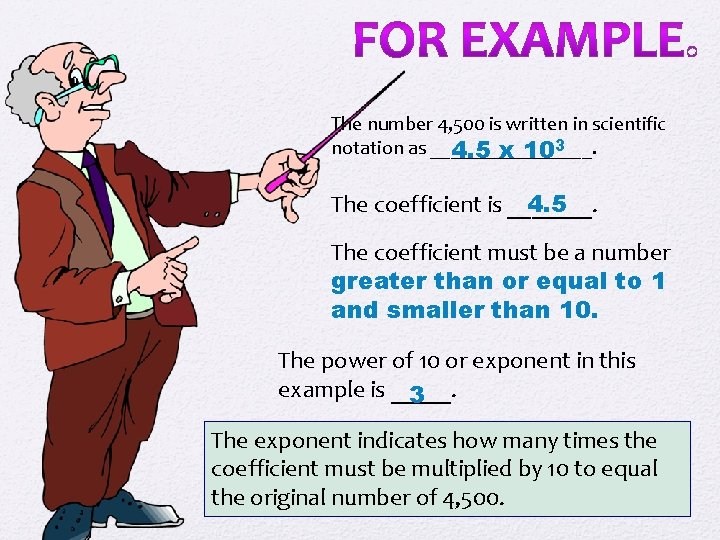The number 4, 500 is written in scientific notation as ________. 4. 5 x 103 The coefficient is _______. 4. 5 The coefficient must be a number greater than or equal to 1 and smaller than 10. The power of 10 or exponent in this example is _____. 3 The exponent indicates how many times the coefficient must be multiplied by 10 to equal the original number of 4, 500.Rules to Remember! If a number is greater than 10, the exponent will be _____ and is equal to positive the number of places the decimal must be moved to right the _____ to write the number in scientific notation.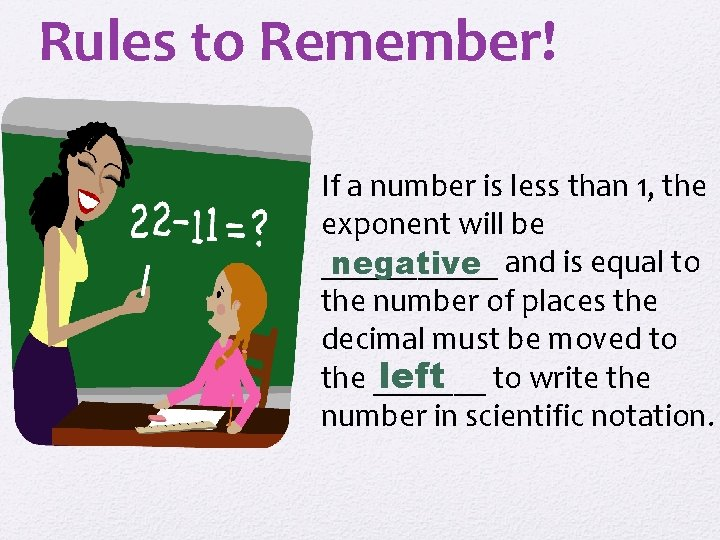Rules to Remember! If a number is less than 1, the exponent will be ______ and is equal to negative the number of places the decimal must be moved to the _______ to write the left number in scientific notation.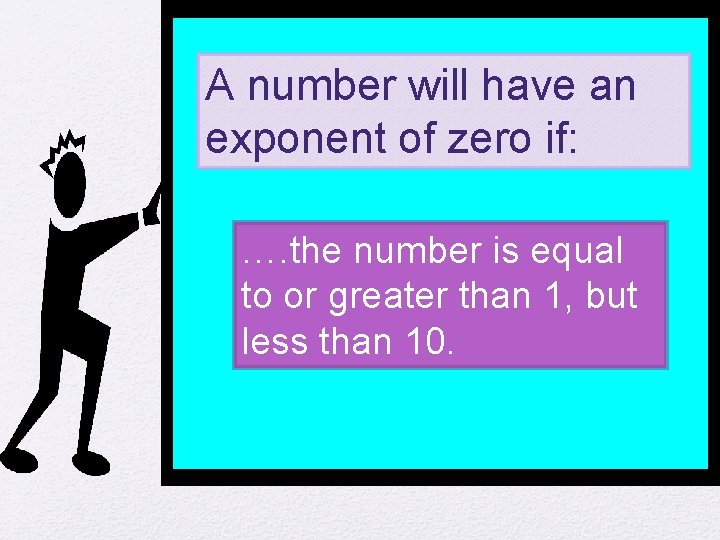A number will have an exponent of zero if: …. the number is equal to or greater than 1, but less than 10.To write a number in scientific notation: 1. Move the decimal to the right of the first non-zero number. 2. Count how many places the decimal had to be moved. 3. If the decimal had to be moved to the right, the exponent is positive 4. If the decimal had to be moved to the left, the exponent is negative. To emphasize again: The exponent counts how many places you move the decimal to the left or right.Practice Problems Express the following in scientific notation. PROBLEMS: 1) 2) 3) 4) 5) 6) 0. 00012 1000 0. 01 12 0. 987 596 ANSWERS 1) 1. 2 x 10 -4 2) 1 x 103 3) 1 x 10 -2 4) 1. 2 x 101 5) 9. 87 x 10 -1 6) 5. 96 x 102Practice Problems Express the following in scientific notation. PROBLEMS: 7) 0. 000 7 8) 1, 000 9) 0. 001257 10) 987, 653, 000 11) 8 ANSWERS 7. 7. 0 x 10 -7 8. 1. 0 x 106 9. 1. 26 x 10 -3 10. 9. 88 x 1011 11. 8 x 100EXPRESS THE FOLLOWING AS WHOLE NUMBERS OR AS DECIMALS PROBLEMS 1) 4. 9 X 102 2) 3. 75 X 10 -2 3) 5. 95 X 10 -4 4) 9. 46 X 103 5) 3. 87 X 101 6) 7. 10 X 100 7) 8. 2 X 10 -5 ANSWERS 1)490 2). 0375 3). 000595 4)9460 5)38. 7 6)7. 10 7). 000082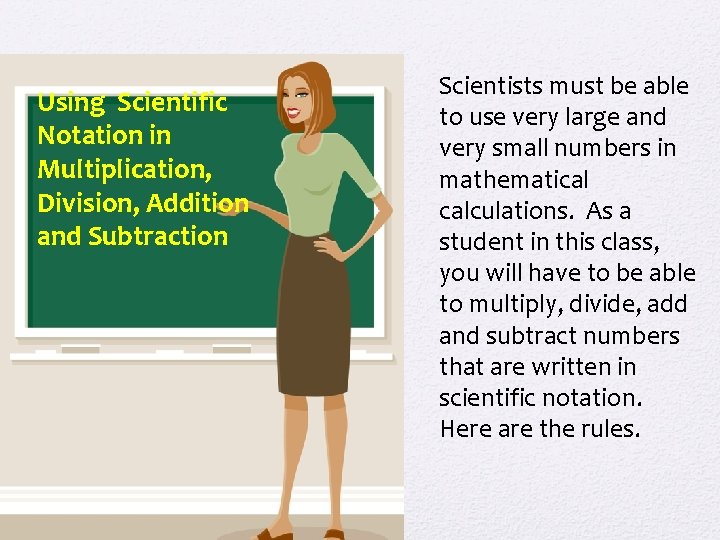Using Scientific Notation in Multiplication, Division, Addition and Subtraction Scientists must be able to use very large and very small numbers in mathematical calculations. As a student in this class, you will have to be able to multiply, divide, add and subtract numbers that are written in scientific notation. Here are the rules.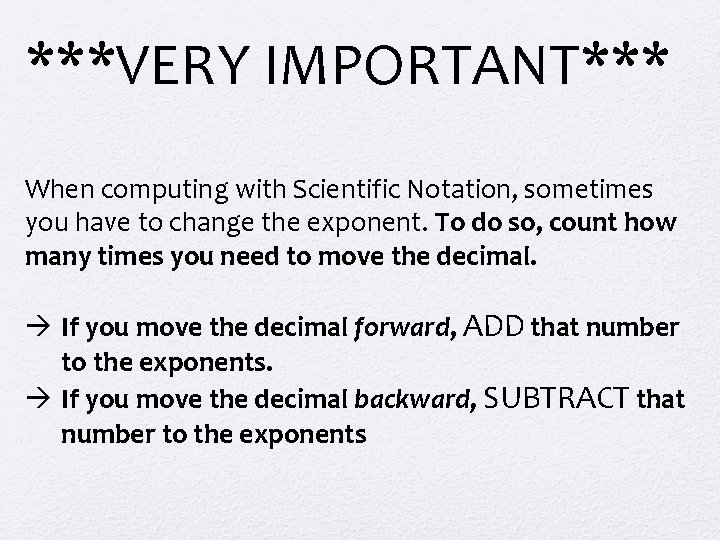***VERY IMPORTANT*** When computing with Scientific Notation, sometimes you have to change the exponent. To do so, count how many times you need to move the decimal. à If you move the decimal forward, ADD that number to the exponents. à If you move the decimal backward, SUBTRACT that number to the exponents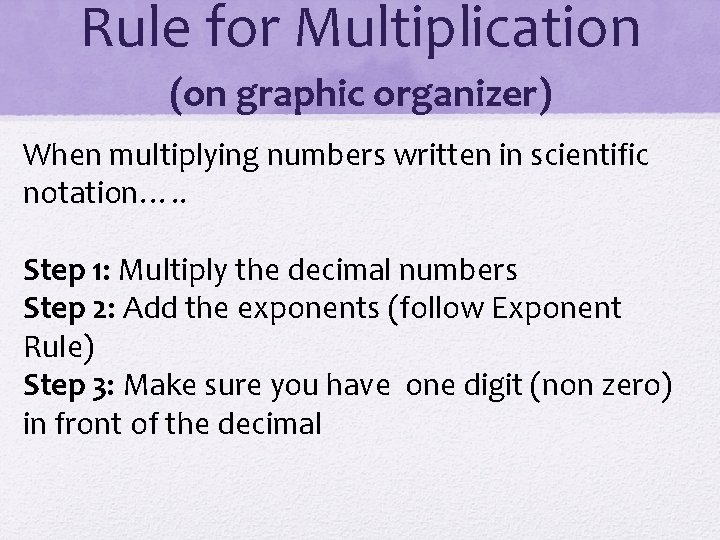Rule for Multiplication (on graphic organizer) When multiplying numbers written in scientific notation…. . Step 1: Multiply the decimal numbers Step 2: Add the exponents (follow Exponent Rule) Step 3: Make sure you have one digit (non zero) in front of the decimalExample (write all steps on Graphic organizer next to the written steps)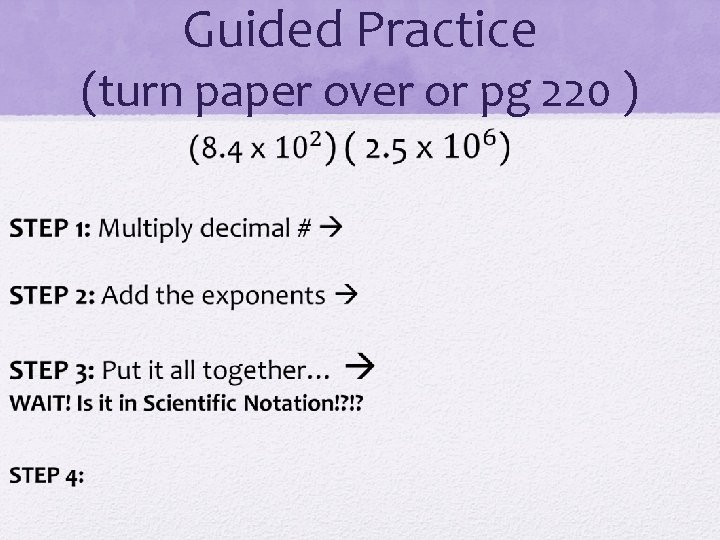Guided Practice (turn paper over or pg 220 )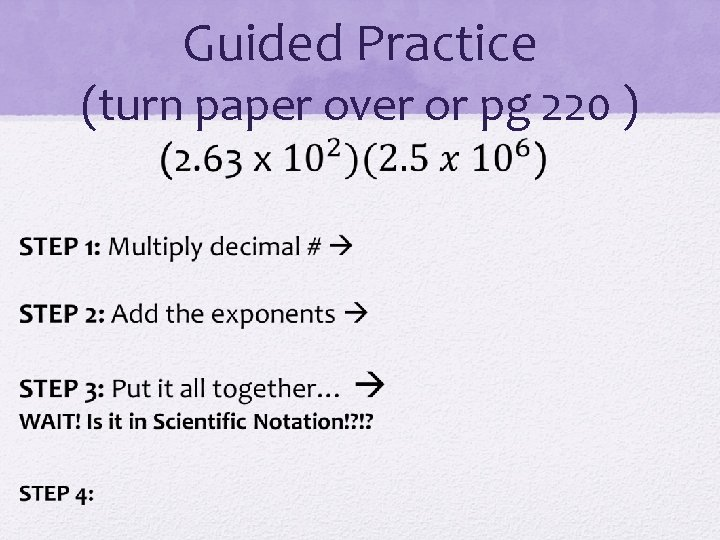Guided Practice (turn paper over or pg 220 )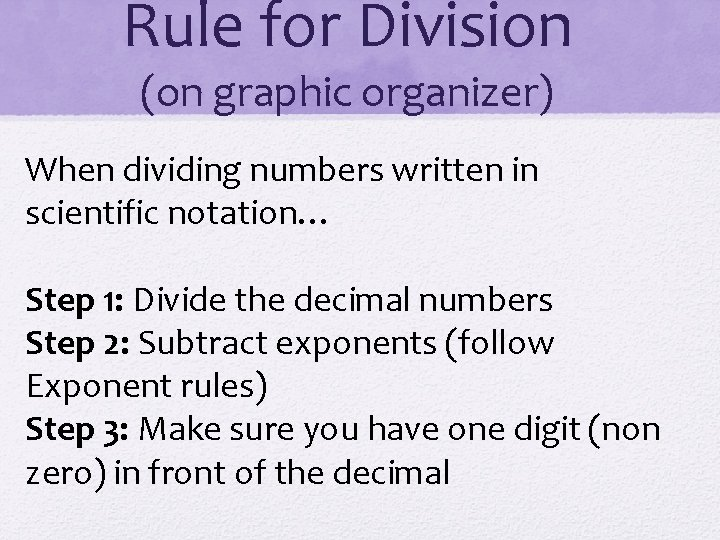Rule for Division (on graphic organizer) When dividing numbers written in scientific notation… Step 1: Divide the decimal numbers Step 2: Subtract exponents (follow Exponent rules) Step 3: Make sure you have one digit (non zero) in front of the decimal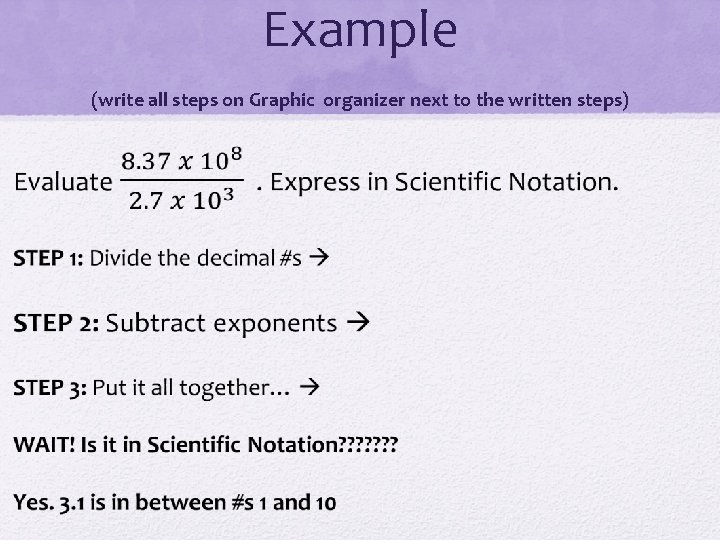Example (write all steps on Graphic organizer next to the written steps)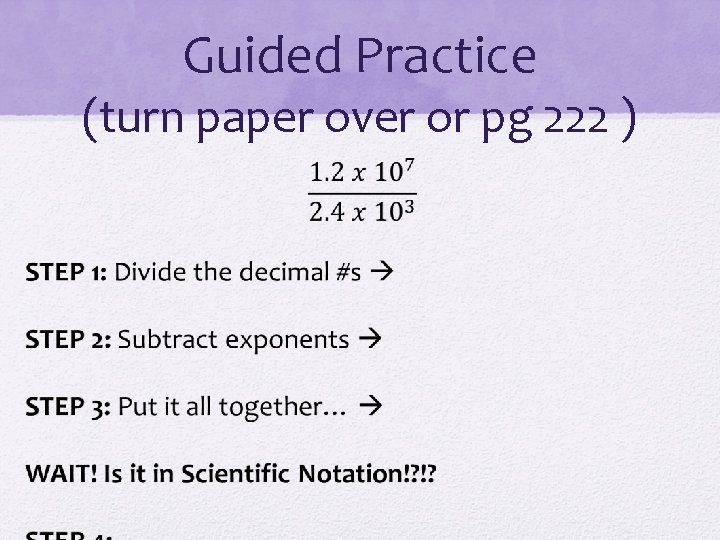Guided Practice (turn paper over or pg 222 )Guided Practice (turn paper over )Classwork: Complete worksheet. Will check for accuracyHW pg 223 EVEN #sRule for Addition and Subtraction (on Graphic Organizer) To add or subtract numbers written in scientific notation, you must…. express them with the same power of ten. Step 1: Make sure that both exponents are the SAME! ** you might have to move a decimal forward or backward to have same exponent #s Step 2: Add decimal #’s together Step 3: Make sure it is in SCIENTIFIC NOTATION!Example: Graphic Organizer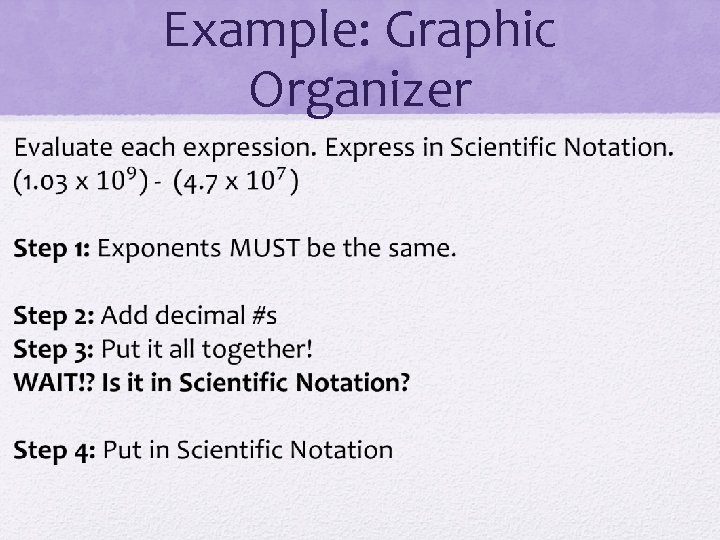Example: Graphic Organizer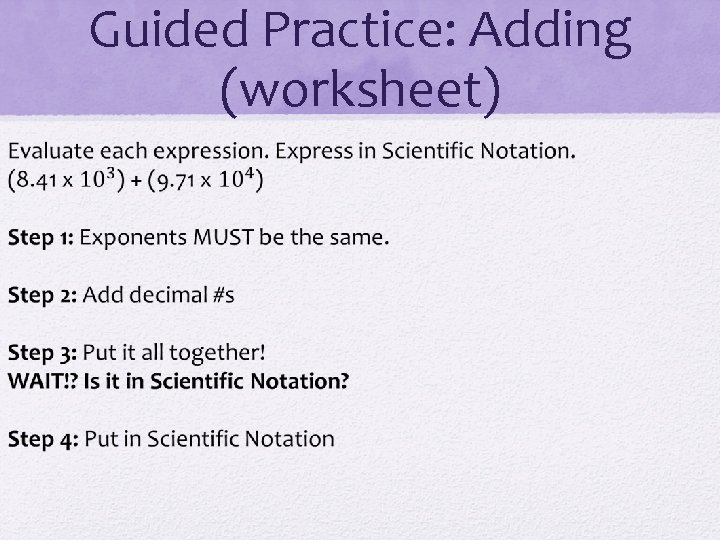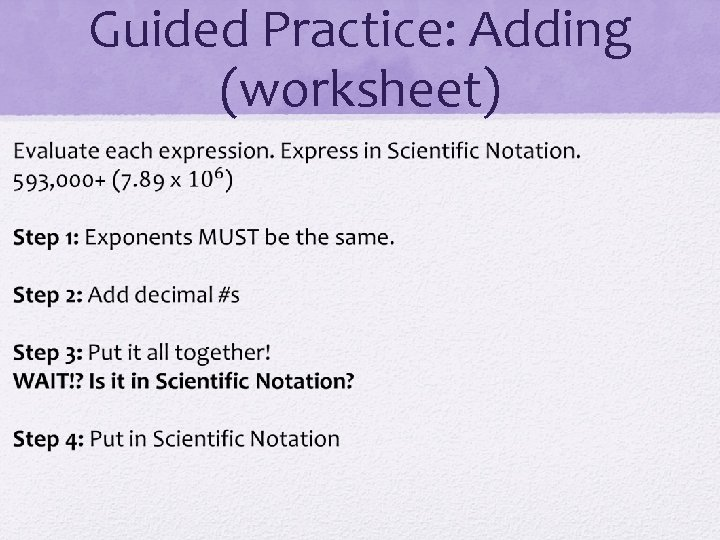Guided Practice: Subtracting (worksheet)Guided Practice: Subtracting (worksheet)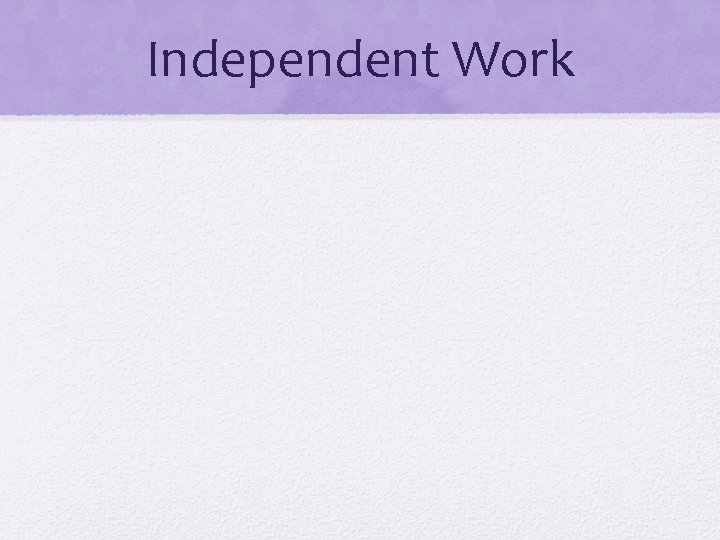Independent Work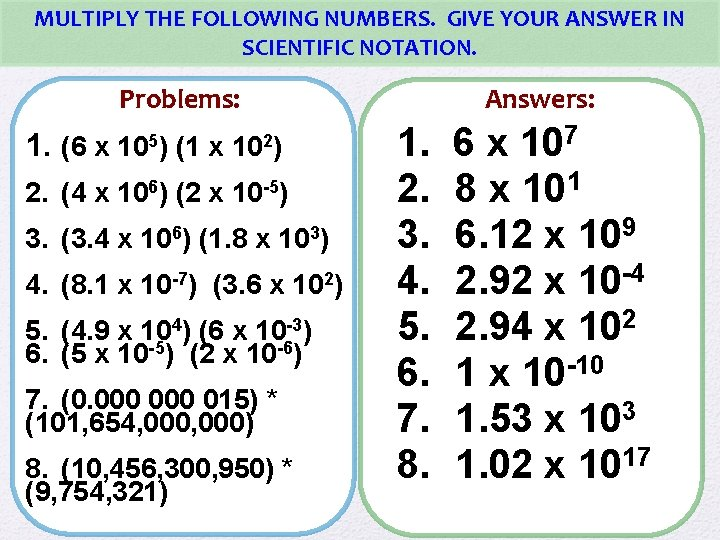MULTIPLY THE FOLLOWING NUMBERS. GIVE YOUR ANSWER IN SCIENTIFIC NOTATION. Problems: 1. (6 x 105) (1 x 102) 2. (4 x 106) (2 x 10 -5) 3. (3. 4 x 106) (1. 8 x 103) 4. (8. 1 x 10 -7) (3. 6 x 102) 5. (4. 9 x 104) (6 x 10 -3) 6. (5 x 10 -5) (2 x 10 -6) 7. (0. 000 015) * (101, 654, 000) 8. (10, 456, 300, 950) * (9, 754, 321) Answers: 1. 6 x 107 2. 8 x 101 3. 6. 12 x 109 4. 2. 92 x 10 -4 5. 2. 94 x 102 6. 1 x 10 -10 7. 1. 53 x 103 8. 1. 02 x 1017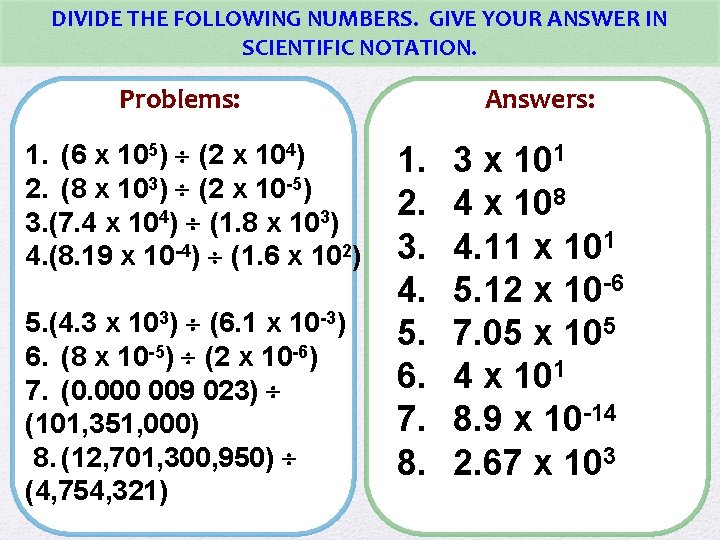DIVIDE THE FOLLOWING NUMBERS. GIVE YOUR ANSWER IN SCIENTIFIC NOTATION. Problems: 1. (6 x 105) (2 x 104) 2. (8 x 103) (2 x 10 -5) 3. (7. 4 x 104) (1. 8 x 103) 4. (8. 19 x 10 -4) (1. 6 x 102) 5. (4. 3 x 103) (6. 1 x 10 -3) 6. (8 x 10 -5) (2 x 10 -6) 7. (0. 000 009 023) (101, 351, 000) 8. (12, 701, 300, 950) (4, 754, 321) Answers: 1. 3 x 101 2. 4 x 108 3. 4. 11 x 101 4. 5. 12 x 10 -6 5. 7. 05 x 105 6. 4 x 101 7. 8. 9 x 10 -14 8. 2. 67 x 103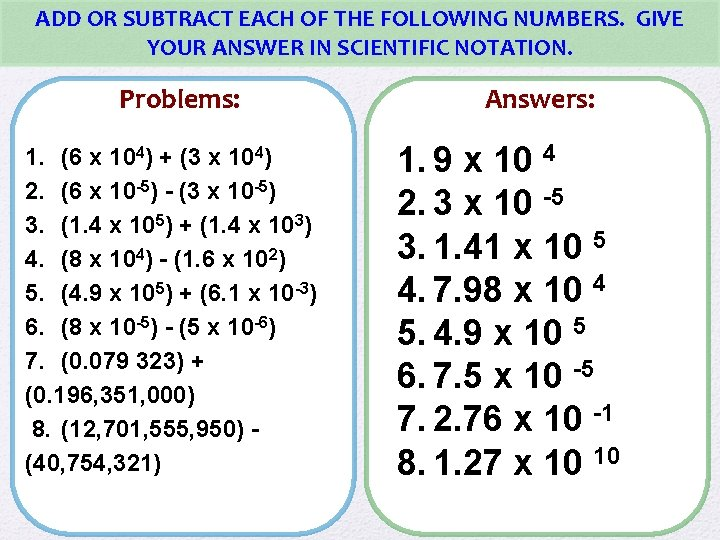ADD OR SUBTRACT EACH OF THE FOLLOWING NUMBERS. GIVE YOUR ANSWER IN SCIENTIFIC NOTATION. Problems: 1. (6 x 104) + (3 x 104) 2. (6 x 10 -5) - (3 x 10 -5) 3. (1. 4 x 105) + (1. 4 x 103) 4. (8 x 104) - (1. 6 x 102) 5. (4. 9 x 105) + (6. 1 x 10 -3) 6. (8 x 10 -5) - (5 x 10 -6) 7. (0. 079 323) + (0. 196, 351, 000) 8. (12, 701, 555, 950) - (40, 754, 321) Answers: 1. 9 x 10 4 2. 3 x 10 -5 3. 1. 41 x 10 5 4. 7. 98 x 10 4 5. 4. 9 x 10 5 6. 7. 5 x 10 -5 7. 2. 76 x 10 -1 8. 1. 27 x 10 10Created by Amy Brown – Science Stuff Copyright © Amy Brown (aka Science Stuff) All rights reserved by author. This document is for your classroom use only. This document may not be electronically distributed or posted to a web site.